Output Devices

For this assigment, we use this Digital control DC power supply to mesure power consumption of an output device.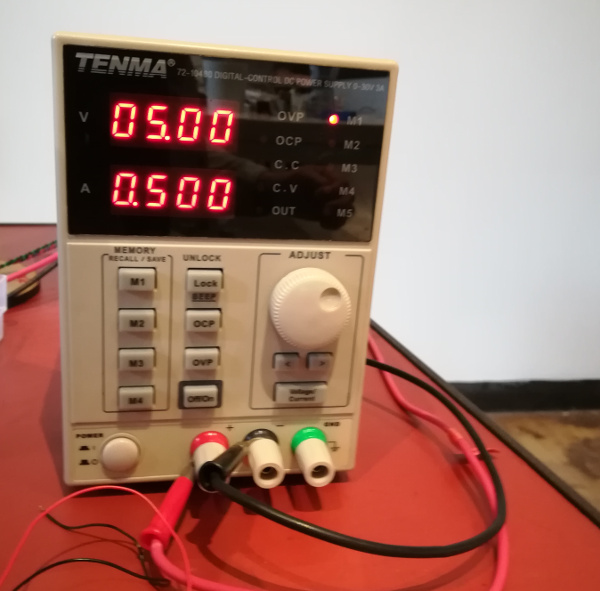We first try with a DC motor. We plug it to the power supply, DC motors are simple: One pin for V, one pin GND: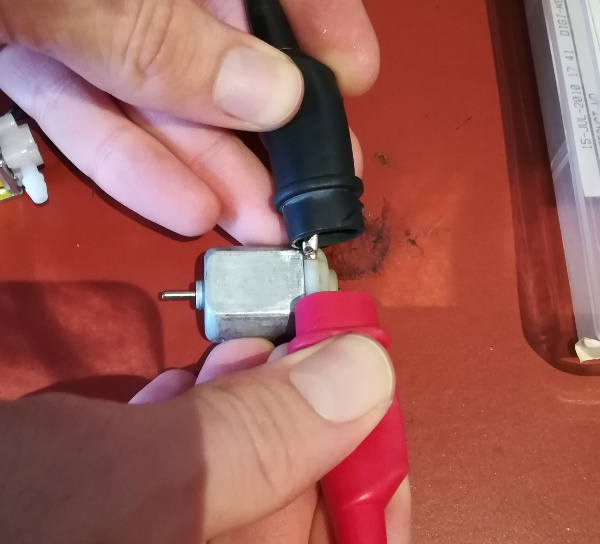Here's the result: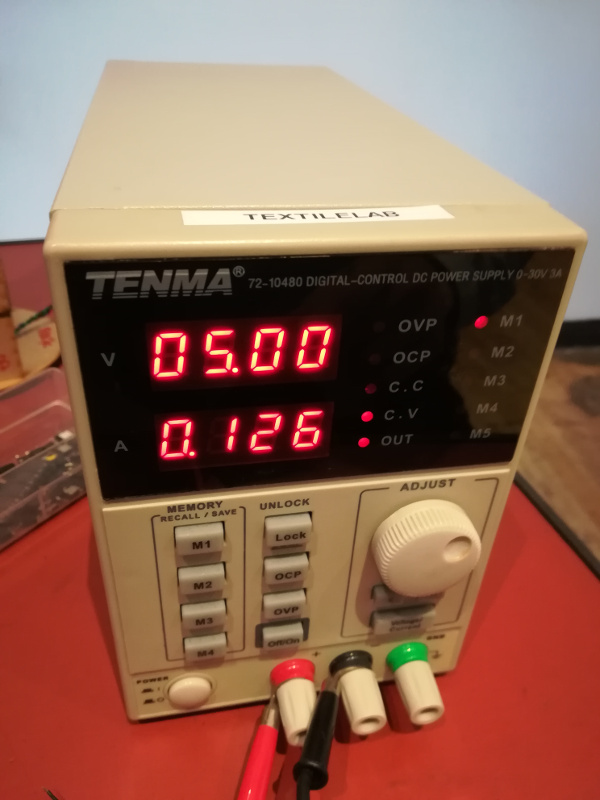We give it 5v and mesure 0.126 A. So power consumption is 5x0.126 = 0.63 W. So 0.63x60 -> 37,8 Wh

We try with an another DC motor: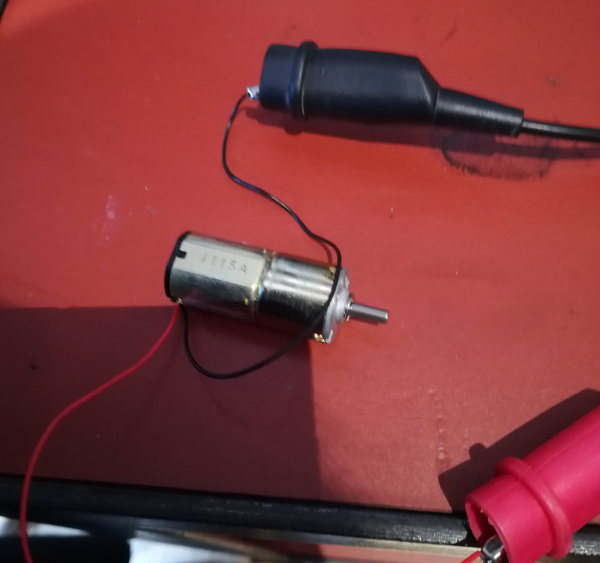Here's the result: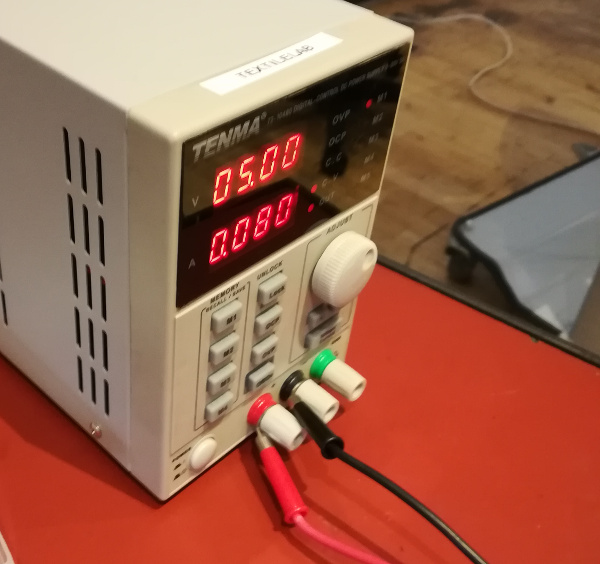We give it 5v and mesure 0.080 A. So power consumption is 5x0.08 = 0.4 W. So 0.4x60 -> 24 Wh We try also with the air valve: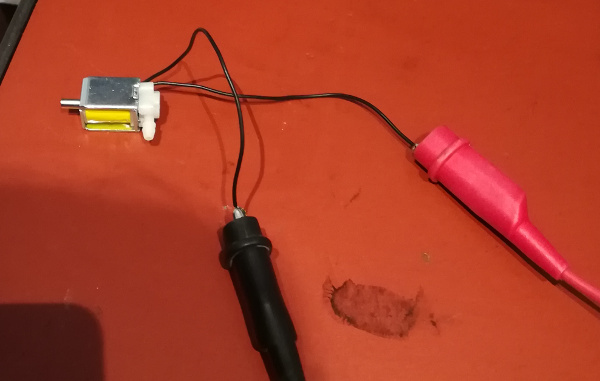Here's the result: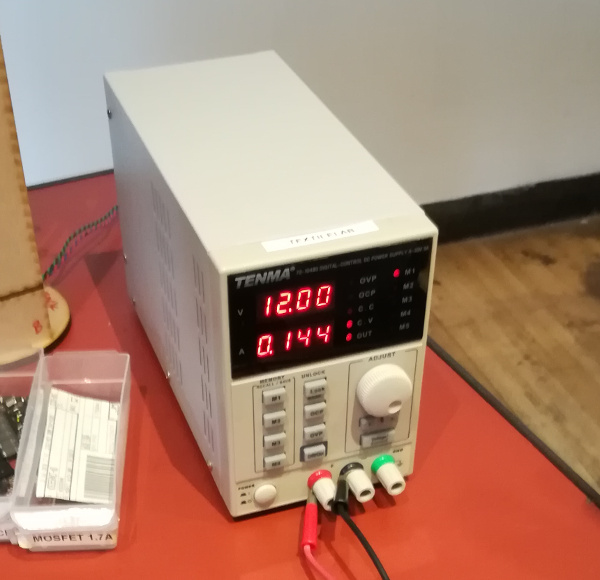We give it 12v and mesure 0.144 A. So power consumption is 12x0.144 = 1.728 W. So 1.728x60 -> 103.68 Wh

Conclusion
DC motor 1, power consumption: 37,8 Wh
DC motor 2, power consumption: 24 Wh
Air valve, power consumption: 103,68 Wh